# K-Means Clustering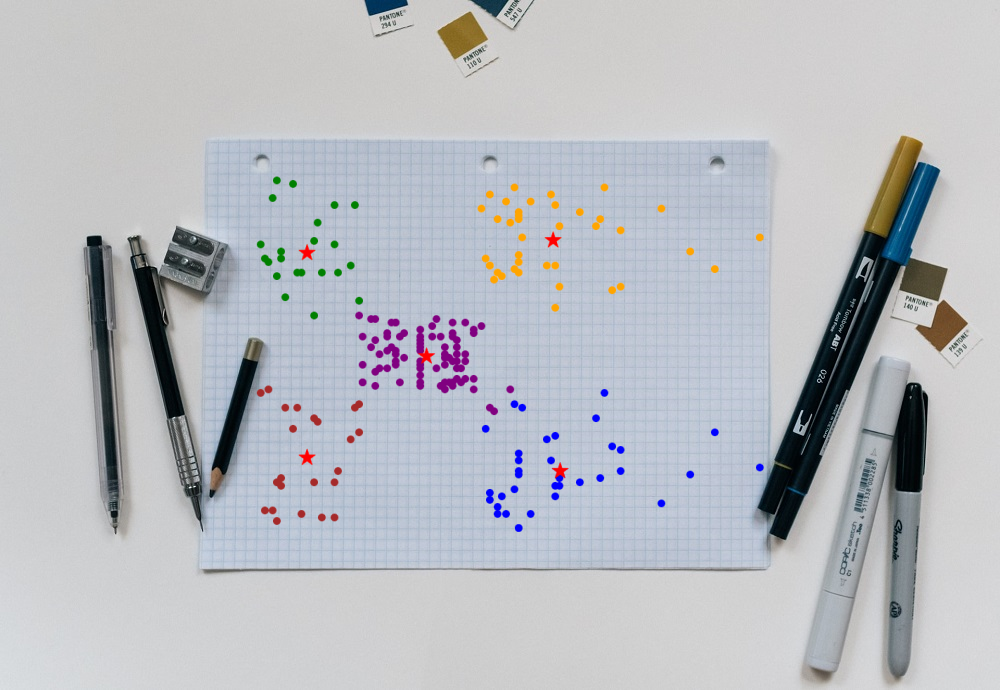# Introduction

If a dataset do not have any labels associated with it, then unsupervised algorithms are used to find some structure in it. These structures can be different types of data pattern or group of data. K-Means clustering is most commonly used unsupervised learning algorithm to find groups in unlabeled data. Here K represents the number of groups or clusters and the process of creating these groups is known as ‘clustering’, that why the name K-means clustering.

# Uses

• Search engine: Search engine, groups results together using clustering algorithm
• Customer segmentation: K-mean clustering can be used to create customer clusters based on demographic information, geographical information and behavioral data.
• Social network analysis: To find groups of people with specific interest to direct the personalized ads.
• Data center: To organize the computer clusters in data center.
• Inventory management: Create inventory clusters based on sales number and manufacturing capacity

# Inner Working of K-Means Clustering

K-means is often referred to as Lloyd’s algorithm. It is one of the most popular clustering algorithm. Refer below plot where there are two clusters (K=2) one is of red data points and another one of green data points.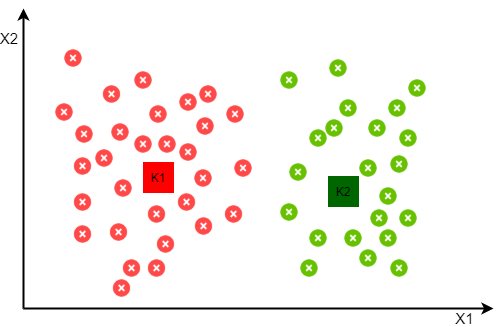So how does K-Means algorithm find the clusters of the data points without any label? Below steps will explain the inner working of K-Means algorithm.

• First step is to finalize the number of clusters you want to identify in your data. This is the “K” in K-means clustering.
• Now randomly initialize the points equal to the number of clusters K. ‘Cluster Centroid’ is the terminology used to refer these points.
• Note that centroid means center point of given dataset, but initially these points are at random location, but at the end when K-Means algorithm will converge they will be at the center of their respective cluster.
• Once cluster centroids are defined, K-means algorithm will go through each data point from given data and depending on that points closeness to cluster centroid, it will assign the data point to the cluster centroid. This is called as ‘Assignment Step’.
• In order to move the cluster centroids from random location to their respective group, K-means algorithm will find the mean of each data point assigned to the cluster centroid and move the respective centroid to the mean value location. This is called as ‘Move Centroid Step’
• Note that during ‘Move Centroid Step’ data points can get reassigned from one cluster to another as centroid position change.
• Now repeat the assignment and move centroid steps till cluster centroid position don’t change. K-means algorithm will converge when we get the unchanged position of cluster centroids.
• Once K-means algorithm is converged, data point assigned to respective centroid will represent the respective cluster.
• During cluster assignment step if we found a centroid who has no data point associated with it, then it’s better to remove it.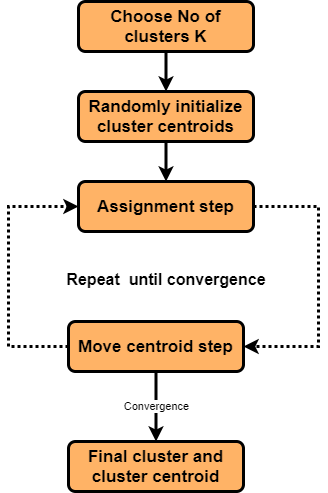Since we have to randomly pick the cluster centroids, it’s initialization may affect the final outcome of the clustering. In case our initialization is not correct, then K-Means algorithm may form a cluster with few points only. Such situation is referred as ‘centroid random initialization trap’ and it may cause algorithm to get stuck at local optima.

Check below plots, where for same dataset, we end up getting different clusters depending on initial position of cluster centroids. Gray color squares represent the initial positions of centroids and red, green and blue squares represent the final position of centroids.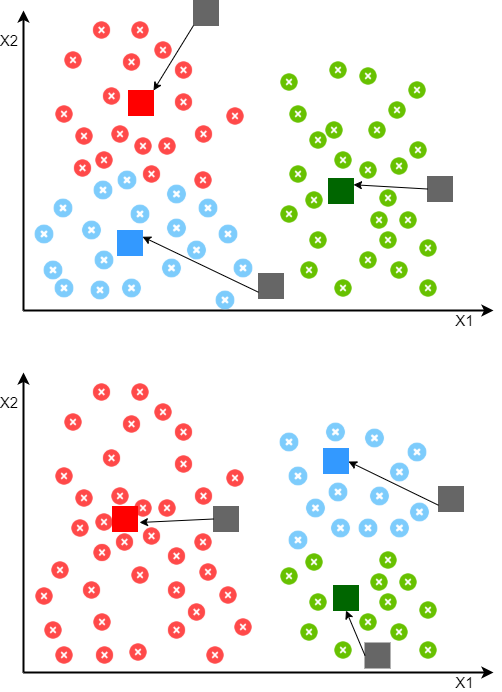# Random Initialization Guidelines

To avoid random initialization trap, follow below guidelines for random initialization.

• Number of cluster centroids should be less than number of training examples
• To avoid local optima issue, try to do multiple random initialization of centroids.
• Multiple random initialization technique is more effective when we have a small number of clusters.
• Similarly for large number of clusters, few random initialization are sufficient

# Choosing The Number of Clusters

So using random initialization we can avoid the local optima issue, but to choose how many clusters to look for in a given data we can use below methods.

## Visualization

To find the number of clusters manually by data visualization is one of the most common method. Domain knowledge and proper understanding of given data also help to make more informed decisions. Since its manual exercise there is always a scope for ambiguous observations, in such cases we can also use ‘Elbow Method’

## Elbow Method

In Elbow method we run the K-Means algorithm multiple times over a loop, with an increasing number of cluster choice(say from 1 to 10) and then plotting a clustering score as a function of the number of clusters. Clustering score is nothing but sum of squared distances of samples to their closest cluster center. Elbow is the point on the plot where clustering score (distortion) slows down, and the value of cluster at that point gives us the optimum number of clusters to have. But sometimes we don’t get clear elbow point on the plot, in such cases its very hard to finalize the number of clusters.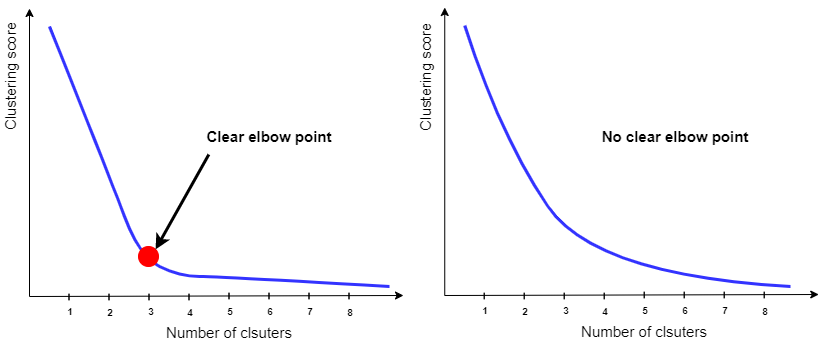# Advantages

• One of the simplest algorithm to understand
• Since it uses simple computations it is relatively efficient
• Gives better results when there is less data overlapping

# Disadvantages

• Number of clusters need to be defined by user
• Doesn’t work well in case of overlapping data
• Unable to handle the noisy data and outliers
• Algorithm fails for non-linear data set

# Python Example

Now we will use k-means clustering algorithm on mall customer unlabeled data to create groups of the customer based on annual spending and spending score assigned by the mall. We are going to use sklearns library for it.

Feel free to copy and edit my kaggle kernel to test below code.

## Import The Library

• pandas: Used for data manipulation and analysis
• numpy : Numpy is the core library for scientific computing in Python. It is used for working with arrays and matrices.
• matplotlib : It’s plotting library, and we are going to use it for data visualization
• KMeans: Sklearn library for K-Means clustering
``````import pandas as pd
import numpy as np
import matplotlib.pyplot as plt
from sklearn.cluster import KMeans
``````

## Load Data

• We are going to use ‘Mall_Customers.csv’ CSV file
• Dataset contains 5 columns CustomerID, Gender, Age, Annual Income (k\$), Spending Score (1-100)
``````#df = pd.read_csv('https://raw.githubusercontent.com/satishgunjal/datasets/master/Mall_Customers.csv')
df = pd.read_csv('/kaggle/input/customer-segmentation-tutorial-in-python/Mall_Customers.csv')
print("Shape of the data= ", df.shape)
df.head()
``````
``````Shape of the data=  (200, 5)
``````
CustomerID Gender Age Annual Income (k\$) Spending Score (1-100)
0 1 Male 19 15 39
1 2 Male 21 15 81
2 3 Female 20 16 6
3 4 Female 23 16 77
4 5 Female 31 17 40

## Understanding The Data

• There are total 200 training example without any label to indicate which customer belong which group
• We are going to use annual income and spending score to find the clusters in data. Note that spending score is from 1 to 100 which is assigned by the mall based on customer behavior and spending nature
``````plt.figure(figsize=(10,6))
plt.scatter(df['Annual Income (k\$)'],df['Spending Score (1-100)'])
plt.xlabel('Annual Income')
plt.ylabel('Spending Score')
plt.title('Unlabelled Mall Customer Data')
``````
``````Text(0.5, 1.0, 'Unlabelled Mall Customer Data')
``````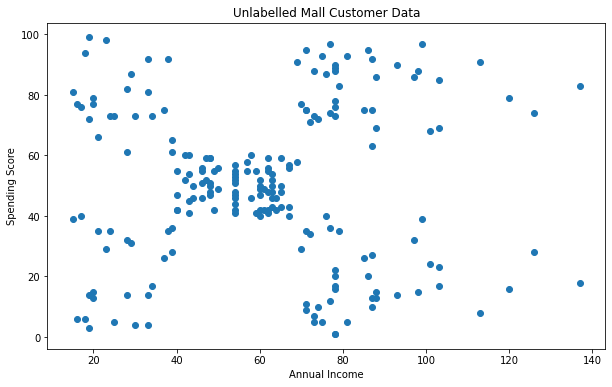``````# Since we are going to use Annual Income and Spending Score  columns only, lets create 2D array of these columns for further use
X = df.iloc[:, [3,4]].values
X[:5] # Show first 5 records only
``````
``````array([[15, 39],
[15, 81],
[16,  6],
[16, 77],
[17, 40]])
``````

## Choosing The Number of Clusters

By visual inspection of above scatter plot, we can identify 5 possible clusters. But since there is no other information available its very difficult say it with 100% confidence. So lets try to verify this with Elbow method technique.

### Elbow Method

• Using the elbow method to find the optimal number of clusters. Let’s use 1 to 11 as range of clusters.
• We will use ‘random’ initialization method for this study.
• Note that Sklearn K-Means algorithm also have ‘k-means++’ initialization method. It selects initial cluster centers for k-mean clustering in a smart way to speed up convergence.
``````clustering_score = []
for i in range(1, 11):
kmeans = KMeans(n_clusters = i, init = 'random', random_state = 42)
kmeans.fit(X)
clustering_score.append(kmeans.inertia_) # inertia_ = Sum of squared distances of samples to their closest cluster center.

plt.figure(figsize=(10,6))
plt.plot(range(1, 11), clustering_score)
plt.scatter(5,clustering_score, s = 200, c = 'red', marker='*')
plt.title('The Elbow Method')
plt.xlabel('No. of Clusters')
plt.ylabel('Clustering Score')
plt.show()
``````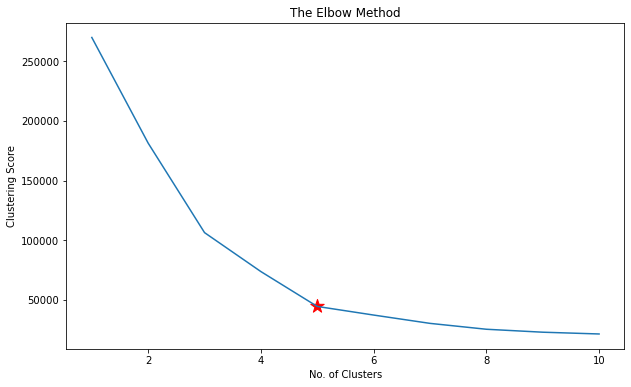From above elbow plot its clear that clustering scores slows down after 5 number of clusters. So we can use K= 5 for further analysis.

## Compute K-Means Clustering

Compute cluster centers and predict cluster index for each sample. Since K=5 we will get the cluster index from 0 to 4 for every data point in our dataset.

``````kmeans= KMeans(n_clusters = 5, random_state = 42)

# Compute k-means clustering
kmeans.fit(X)

# Compute cluster centers and predict cluster index for each sample.
pred = kmeans.predict(X)

pred
``````
``````array([3, 0, 3, 0, 3, 0, 3, 0, 3, 0, 3, 0, 3, 0, 3, 0, 3, 0, 3, 0, 3, 0,
3, 0, 3, 0, 3, 0, 3, 0, 3, 0, 3, 0, 3, 0, 3, 0, 3, 0, 3, 0, 3, 1,
3, 0, 1, 1, 1, 1, 1, 1, 1, 1, 1, 1, 1, 1, 1, 1, 1, 1, 1, 1, 1, 1,
1, 1, 1, 1, 1, 1, 1, 1, 1, 1, 1, 1, 1, 1, 1, 1, 1, 1, 1, 1, 1, 1,
1, 1, 1, 1, 1, 1, 1, 1, 1, 1, 1, 1, 1, 1, 1, 1, 1, 1, 1, 1, 1, 1,
1, 1, 1, 1, 1, 1, 1, 1, 1, 1, 1, 1, 1, 2, 4, 2, 1, 2, 4, 2, 4, 2,
1, 2, 4, 2, 4, 2, 4, 2, 4, 2, 1, 2, 4, 2, 4, 2, 4, 2, 4, 2, 4, 2,
4, 2, 4, 2, 4, 2, 4, 2, 4, 2, 4, 2, 4, 2, 4, 2, 4, 2, 4, 2, 4, 2,
4, 2, 4, 2, 4, 2, 4, 2, 4, 2, 4, 2, 4, 2, 4, 2, 4, 2, 4, 2, 4, 2,
4, 2], dtype=int32)
``````

‘pred’ contains the values index( 0 to 4) cluster for every training example. Let’s add it to original dataset for better understanding.

``````df['Cluster'] = pd.DataFrame(pred, columns=['cluster'] )
print('Number of data points in each cluster= \n', df['Cluster'].value_counts())
df
``````
``````Number of data points in each cluster=
1    81
2    39
4    35
3    23
0    22
Name: Cluster, dtype: int64
``````
CustomerID Gender Age Annual Income (k\$) Spending Score (1-100) Cluster
0 1 Male 19 15 39 3
1 2 Male 21 15 81 0
2 3 Female 20 16 6 3
3 4 Female 23 16 77 0
4 5 Female 31 17 40 3
... ... ... ... ... ... ...
195 196 Female 35 120 79 2
196 197 Female 45 126 28 4
197 198 Male 32 126 74 2
198 199 Male 32 137 18 4
199 200 Male 30 137 83 2

200 rows × 6 columns

## Visualization

Let’s plot the centroid and cluster with different colors to visualize, how K-Means algorithm has grouped the data.

``````plt.figure(figsize=(10,6))
plt.scatter(X[pred == 0, 0], X[pred == 0, 1], c = 'brown', label = 'Cluster 0')
plt.scatter(X[pred == 1, 0], X[pred == 1, 1], c = 'green', label = 'Cluster 1')
plt.scatter(X[pred == 2, 0], X[pred == 2, 1], c = 'blue', label = 'Cluster 2')
plt.scatter(X[pred == 3, 0], X[pred == 3, 1], c = 'purple', label = 'Cluster 3')
plt.scatter(X[pred == 4, 0], X[pred == 4, 1], c = 'orange', label = 'Cluster 4')

plt.scatter(kmeans.cluster_centers_[:,0], kmeans.cluster_centers_[:, 1],s = 300, c = 'red', label = 'Centroid', marker='*')

plt.xlabel('Annual Income')
plt.ylabel('Spending Score')
plt.legend()
plt.title('Customer Clusters')
``````
``````Text(0.5, 1.0, 'Customer Clusters')
``````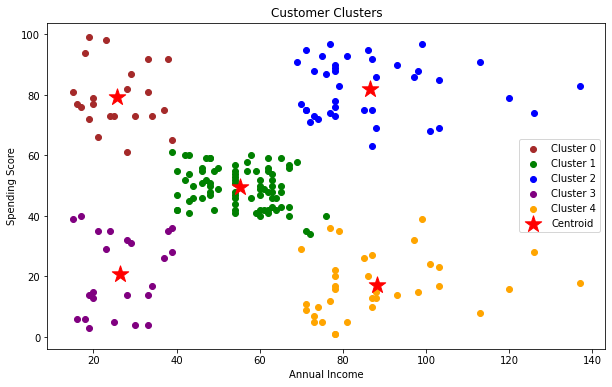## Inner Working

Using below code we can visualize the inner working of K-Means algorithm.

• To start with we will define the random centroids. You can see in below plot that initial centroids with original data without any clusters.
• In step 1 we will run the K-Means algorithm only for one iteration and plot the new position of centroid. Notice how centroid position changes and clusters started to form around it.
• In step 2, we will run the K-Means algorithm for two iterations. Notice how data points are reassigned from one cluster to another as centroid position change
• Similarly at the end we run the K-Means algorithm for six iterations, where we get the final location of centroids and associated clusters.
``````def plot_k_means_progress(centroid_history,n_clusters, centroid_sets, cluster_color):
"""
This function will plot the path taken by the centroids

I/P:
* centroid_history: 2D array of centroids. Each element represent the centroid coordinate.
If there are 5 clusters then first set contains initial cluster cordinates
(i.e. first 5 elements) and then k_means loop will keep appending new cluster coordinates for each iteration
* n_clusters: Total number of clusters to find
* centroid_sets: At the start we set random values as our first centroid set. K-Means loop will keep adding
new centroid sets to centroid_history. Since we are ploting the path of centroid locations, centroid set value
will be K-Means loop iteration number plus 1 for initial centroid set.
So its value will be from 2 to K-Means loops max iter plus 1
* cluster_color: Just to have same line and cluster color

O/P: Plot the centroid path
"""
c_x = [] # To store centroid X coordinated
c_y=[]   # To store the centroid Y coordinates
for i in range(0, n_clusters):
cluster_index = 0
for j in range(0, centroid_sets):
c_x = np.append(c_x, centroid_history[:,0][i + cluster_index])
c_y = np.append(c_y, centroid_history[:,1][i + cluster_index])
cluster_index = cluster_index + n_clusters
# if there are 5 clusters then first set contains initial cluster cordinates and then k_means loop will keep appending new cluster coordinates for each iteration

plt.plot(c_x, c_y, c= cluster_color['c_' + str(i)], linestyle='--')

# Reset coordinate arrays to avoid continuous lines
c_x = []
c_y=[]
``````
``````plt.figure(figsize=(10,6))

# Random Initialization of Centroids
plt.scatter(df['Annual Income (k\$)'],df['Spending Score (1-100)'])
initial_centroid = np.array([[10, 2], [50,100], [130,20], [50,15], [140,100]])

plt.scatter(initial_centroid[:,0], initial_centroid[:, 1],s = 200, c = 'red', label = 'Random Centroid', marker='*')
plt.xlabel('Annual Income')
plt.ylabel('Spending Score')
plt.legend()
plt.title('Random Initialization of Centroids')

# K-Means loop of assignment and move centroid steps
centroid_history = []
centroid_history = initial_centroid
#
cluster_color= {'c_0':'brown','c_1':'green','c_2':'blue','c_3':'purple','c_4':'orange'}
n_clusters = 5
for i in range(1,6):
kmeans= KMeans(n_clusters, init= initial_centroid, n_init= 1, max_iter= i, random_state = 42)  #n_init= 1 since our init parameter is array

# Compute cluster centers and predict cluster index for each sample
pred = kmeans.fit_predict(X)

plt.figure(figsize=(10,6))
plt.scatter(X[pred == 0, 0], X[pred == 0, 1], c = 'brown', label = 'Cluster 0')
plt.scatter(X[pred == 1, 0], X[pred == 1, 1], c = 'green', label = 'Cluster 1')
plt.scatter(X[pred == 2, 0], X[pred == 2, 1], c = 'blue', label = 'Cluster 2')
plt.scatter(X[pred == 3, 0], X[pred == 3, 1], c = 'purple', label = 'Cluster 3')
plt.scatter(X[pred == 4, 0], X[pred == 4, 1], c = 'orange', label = 'Cluster 4')

plt.scatter(centroid_history[:,0], centroid_history[:, 1],s = 50, c = 'gray', label = 'Last Centroid', marker='x')

plt.scatter(kmeans.cluster_centers_[:,0], kmeans.cluster_centers_[:, 1],s = 200, c = 'red', label = 'Centroid', marker='*')

centroid_history = np.append(centroid_history, kmeans.cluster_centers_, axis=0)

plt.xlabel('Annual Income')
plt.ylabel('Spending Score')
plt.legend()
plt.title('Iteration:' + str(i) + ' Assignment and Move Centroid Step')

centroid_sets = i + 1 # Adding one for initial set of centroids
plot_k_means_progress(centroid_history,n_clusters, centroid_sets, cluster_color)
``````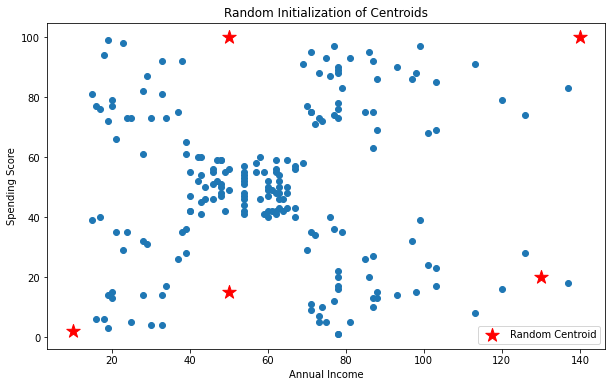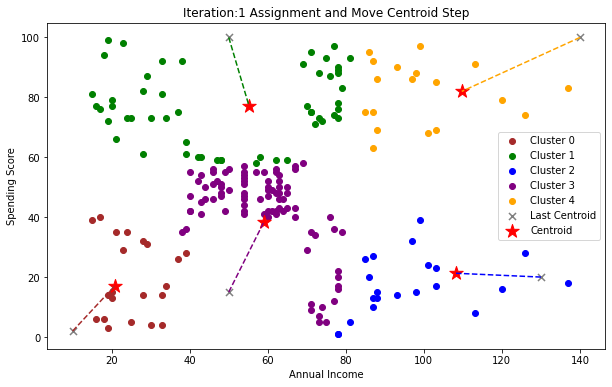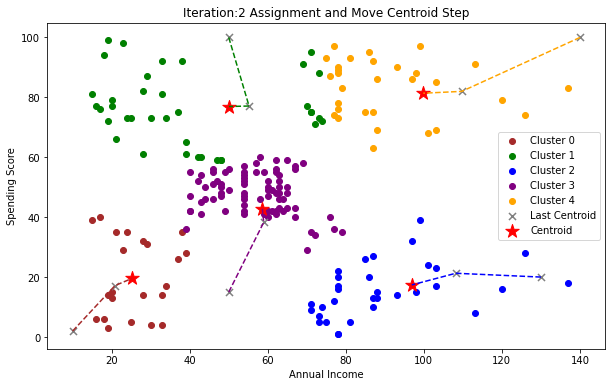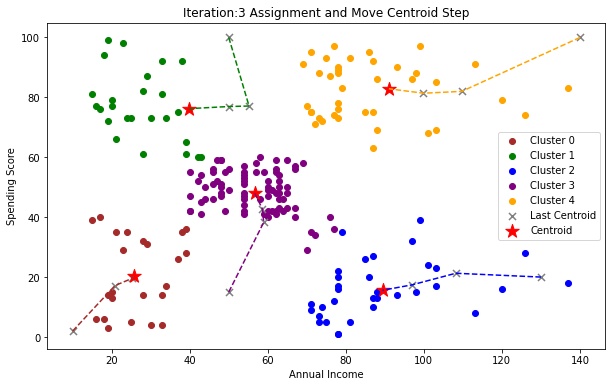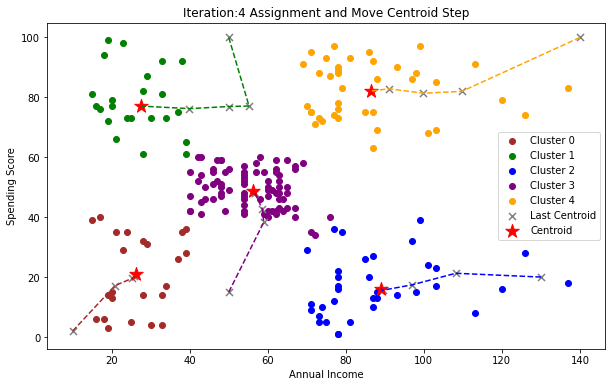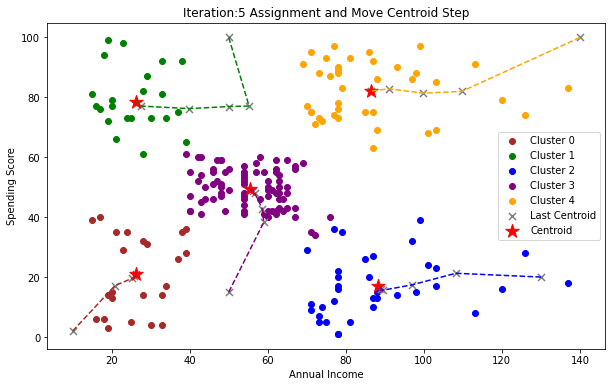# Inner Working: GIF

Isn’t a GIF makes a K-Means clustering visualization even more satisfying!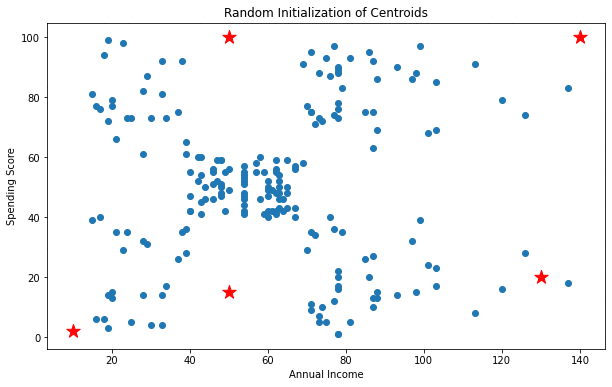Source code used in this article is available at this Kaggle kernel

## Learning Path for DP-900 Microsoft Azure Data Fundamentals Certification

8 minute read

Learning path to gain necessary skills and to clear the Azure Data Fundamentals Certification. This certification is intended for candidates beginning to wor...

## Learning Path for AI-900 Microsoft Azure AI Fundamentals Certification

7 minute read

Learning path to gain necessary skills and to clear the Azure AI Fundamentals Certification. This certification is intended for candidates with both technica...

## ANN Model to Classify Images

12 minute read

In this guide we are going to create and train the neural network model to classify the clothing images. We will use TensorFlow deep learning framework along...

## Introduction to NLP

8 minute read

In short NLP is an AI technique used to do text analysis. Whenever we have lots of text data to analyze we can use NLP. Apart from text analysis, NLP also us...

## K Fold Cross Validation

14 minute read

There are multiple ways to split the data for model training and testing, in this article we are going to cover K Fold and Stratified K Fold cross validation...

## K-Means Clustering

12 minute read

K-Means clustering is most commonly used unsupervised learning algorithm to find groups in unlabeled data. Here K represents the number of groups or clusters...

## Time Series Analysis and Forecasting

10 minute read

Any data recorded with some fixed interval of time is called as time series data. This fixed interval can be hourly, daily, monthly or yearly. Objective of t...

## Support Vector Machines

9 minute read

Support vector machines is one of the most powerful ‘Black Box’ machine learning algorithm. It belongs to the family of supervised learning algorithm. Used t...

## Random Forest

11 minute read

Random forest is supervised learning algorithm and can be used to solve classification and regression problems. Unlike decision tree random forest fits multi...

## Decision Tree

14 minute read

Decision tree explained using classification and regression example. The objective of decision tree is to split the data in such a way that at the end we hav...

## Agile Scrum Framework

7 minute read

This tutorial covers basic Agile principles and use of Scrum framework in software development projects.

## Underfitting & Overfitting

2 minute read

Main objective of any machine learning model is to generalize the learning based on training data, so that it will be able to do predictions accurately on un...

## Multiclass Logistic Regression Using Sklearn

6 minute read

In this study we are going to use the Linear Model from Sklearn library to perform Multi class Logistic Regression. We are going to use handwritten digit’s d...

## Binary Logistic Regression Using Sklearn

5 minute read

In this tutorial we are going to use the Logistic Model from Sklearn library. We are also going to use the same test data used in Logistic Regression From Sc...

## Logistic Regression From Scratch With Python

13 minute read

This tutorial covers basic concepts of logistic regression. I will explain the process of creating a model right from hypothesis function to algorithm. We wi...

## Train Test Split

3 minute read

In this tutorial we are going to study about train, test data split. We will use sklearn library to do the data split.

## One Hot Encoding

11 minute read

In this tutorial we are going to study about One Hot Encoding. We will also use pandas and sklearn libraries to convert categorical data into numeric data.

## Multivariate Linear Regression Using Scikit Learn

8 minute read

In this tutorial we are going to use the Linear Models from Sklearn library. Scikit-learn is one of the most popular open source machine learning library for...

## Univariate Linear Regression Using Scikit Learn

7 minute read

In this tutorial we are going to use the Linear Models from Sklearn library. Scikit-learn is one of the most popular open source machine learning library for...

## Multivariate Linear Regression From Scratch With Python

10 minute read

In this tutorial we are going to cover linear regression with multiple input variables. We are going to use same model that we have created in Univariate Lin...

## Univariate Linear Regression From Scratch With Python

13 minute read

This tutorial covers basic concepts of linear regression. I will explain the process of creating a model right from hypothesis function to gradient descent a...

## Machine Learning Introduction And Learning Plan

4 minute read

In this tutorial we will see the brief introduction of Machine Learning and preferred learning plan for beginners

Back to top ↑